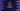# 4 different C# program to find the HCF of two numbers## C# program to find the HCF of two numbers:

In this post, we will learn how to find the HCF of two numbers in C#. HCF or highest common factor of two numbers is the largest number that can divide both of these numbers.

For example, the HCF of 5 and 10 is 5 because 5 is the largest number that can divide both.

### Method 1: By using a for loop to find the HCF:

Let’s use a for loop to find the HCF. The loop will start from 1 and it will run to the smaller number. For each value of the loop, it will check if it can divide both of these numbers or not. If yes, it will assign that value as the HCF. At the end of the loop, the variable to hold the HCF will have the required HCF.

Below is the complete program:

``````namespace Program
{

class Program
{
static int FindHCF(int First, int Second)
{
int Min = First < Second ? First : Second;
int HCF = 1;

for (int i = 1; i <= Min; i++)
{
if (First % i == 0 && Second % i == 0)
{
HCF = i;
}
}
return HCF;
}

static void Main(string[] args)
{
int First, Second, HCF;

Console.WriteLine("Enter the first number:");

Console.WriteLine("Enter the second number:");

HCF = FindHCF(First, Second);

Console.WriteLine("HCF: " + HCF);
}
}
}``````

Here,

• The user input numbers are stored in the integer variables First and Second.
• FindHCF method is called to find the HCF of these two numbers.

• It finds the smaller number and stores it in the Min variable.
• The for loop runs from 1 to Min of i and for each value of i, it checks if the current value of i can divide both numbers or not.
• If yes, it assigns the value of i to the variable HCF.
• At the end of the method, it returns the HCF value.

It will give output as like below:

``````Enter the first number:
5
Enter the second number:
12
HCF: 1

Enter the first number:
9
Enter the second number:
12
HCF: 3``````

### Method 2: By using a while loop:

We can write the above program with a while loop instead of a for loop. It will be almost similar to the above program. Let’s write it with a while loop:

``````namespace Program
{

class Program
{
static int FindHCF(int First, int Second)
{
int Min = First < Second ? First : Second;
int HCF = 1, i = 1;

while (i <= Min)
{
if (First % i == 0 && Second % i == 0)
{
HCF = i;
}
i++;
}
return HCF;
}

static void Main(string[] args)
{
int First, Second, HCF;

Console.WriteLine("Enter the first number:");

Console.WriteLine("Enter the second number:");

HCF = FindHCF(First, Second);

Console.WriteLine("HCF: " + HCF);
}
}
}``````

The only difference we added to this program is to use a while loop instead of a for loop. The while loop checks a condition and if it is true, it executes the code written in the loop block.

It will print similar results.

### Method 3: By changing the larger value repeatedly:

This is another way to find the HCF of two numbers. We will run the following two steps repeatedly until both numbers are equal:

• Subtract the smaller value from the larger one.
• Assign the result to the larger. Once both of these numbers become equal, it will be the required HCF.

For example, if we want to find the HCF of 3 and 11,

• Let’s say x = 3, y = 11
• Subtract 3 from 11 and assign it to y since y > x.
• x = 3, y = 8. Subtract 3 from 8 and assign it to y since y > x.
• x = 3, y = 5. Subtract 3 from 5 and assign it to y since y > x.
• x = 3, y = 2. Subtract 2 from 3 and assign it to x since x > y.
• x = 1, y = 2. Subtract 1 from 2 and assign it to y since y > x.
• x = 1, y = 1.

Since both x and y are equal, 1 is the required HCF.

``````namespace Program
{

class Program
{
static int FindHCF(int First, int Second)
{
while (First != Second)
{
if (First > Second)
First = First - Second;
else
Second = Second - First;
}
return First;
}

static void Main(string[] args)
{
int First, Second, HCF;

Console.WriteLine("Enter the first number:");

Console.WriteLine("Enter the second number:");

HCF = FindHCF(First, Second);

Console.WriteLine("HCF: " + HCF);
}
}
}``````

This program is using the same algorithm we discussed above. The while loop runs until the values of First and Second become equal. Inside the loop, we are updating the value of First or Second and it returns First at the end.

It will print similar result.

### Method 4: Euclidean Algorithm:

There is another way to find the HCF of two numbers. This algorithm is also known as the Euclidean Algorithm. With this algorithm, we will recursively find the HCF of two numbers. The below program uses Euclidean Algorithm to find the HCF:

``````namespace Program
{

class Program
{
static int FindHCF(int First, int Second)
{
if (Second == 0)
return First;
return FindHCF(Second, First % Second);
}

static void Main(string[] args)
{
int First, Second, HCF;

Console.WriteLine("Enter the first number:");